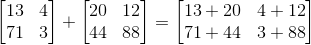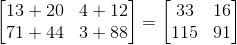## Example Questions

### Example Question #1 : Matrices

Evaluate: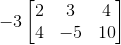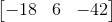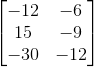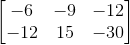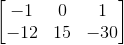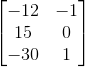Explanation:

This problem involves a scalar multiplication with a matrix. Simply distribute the negative three and multiply this value with every number in the 2 by 3 matrix. The rows and columns will not change.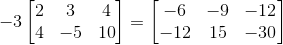### Example Question #2 : Matrices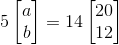What is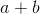?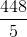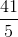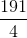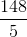Explanation:

You can begin by treating this equation just like it was: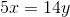That is, you can divide both sides by: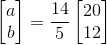Now, for scalar multiplication of matrices, you merely need to multiply the scalar by each component: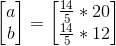Then, simplify: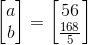Therefore,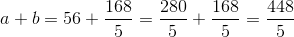### Example Question #3 : Matrices

If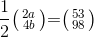, what is?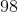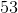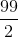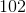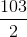Explanation:

Begin by distributing the fraction through the matrix on the left side of the equation. This will simplify the contents, given that they are factors of: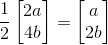Now, this means that your equation looks like: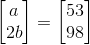This simply means: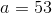and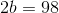or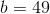Therefore,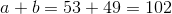### Example Question #4 : Matrices

Simplify: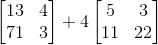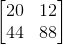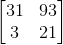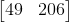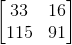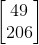Explanation:

Scalar multiplication and addition of matrices are both very easy. Just like regular scalar values, you do multiplication first: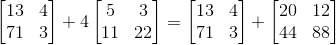The addition of matrices is very easy. You merely need to add them directly together, correlating the spaces directly.